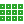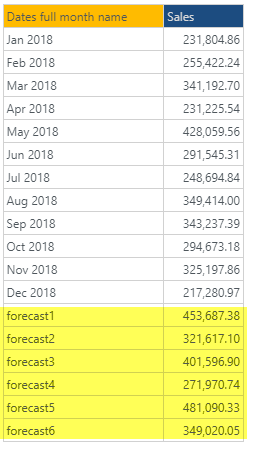#ForecastPercent (PQL)

Returns the percentage change between the last actual and forecasted values for the specified periods using 'best fit'

• Returned Output: Numeric
• Library: PQL \ Dynamic \ Grid
• Version: 2020.02.000
• Compatibility: Any content (regardless of data source) in the relevant parts of the application

#### Syntax

ForecastPercent(, )

* Click on the function's arguments above for more details on the input values.

• The numeric listing is the set of 'existing' values that will drive the forecast formula.
• The forecast periods is the number of periods to forecast into the future,
• Forecasting uses the internal 'best fit' engine that appraises several forecasting algorithms and chooses the one with the highest statistical fit.

#### Examples

The example below uses the following example grid.

Note that the yellow forecasted results are shown to demonstrate the outcomes.This example returns 60.63% - which is the percentage growth of the forecasted value for the 6th future period based on the 2018 data compared to the December 2018 value of 217280.97

ForecastPercent(dataSetColumn(0), 6)